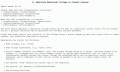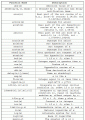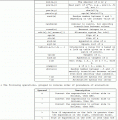# Help Required About PSIM Simulation

#### Majidpk08

Joined Feb 28, 2015
2
Hello All,

I am simulating a 4-phase interleaved buck converter in PSIM. For interleaved multiphase buck converter the output of PWM should be phase shifted among adjacent phases by 360°/N. where N is the number of Phases.

360°/4=90°
for phase 1= PWM Output
for Phase 2= 90° Phase shift of PWM Output
for Phase 3=180° Phase shift of PWM Output
for phase 4=270° Phase shift of PWM Output

my question is how can i implement the phase shift function in PSIM. Any help regarding this will be highly appreciated.

#### MikeML

Joined Oct 2, 2009
5,444
Does PSim have a Behavioral Voltage Source?

This would be trivial to do in LTSpice using the BV (behavioral voltage source), using the delay() function shown in the following extract from LTSpice's Help File.Check out the list of other library functions that can easily be included in any running LTSpice simulation...

•Majidpk08